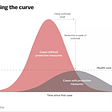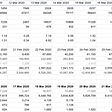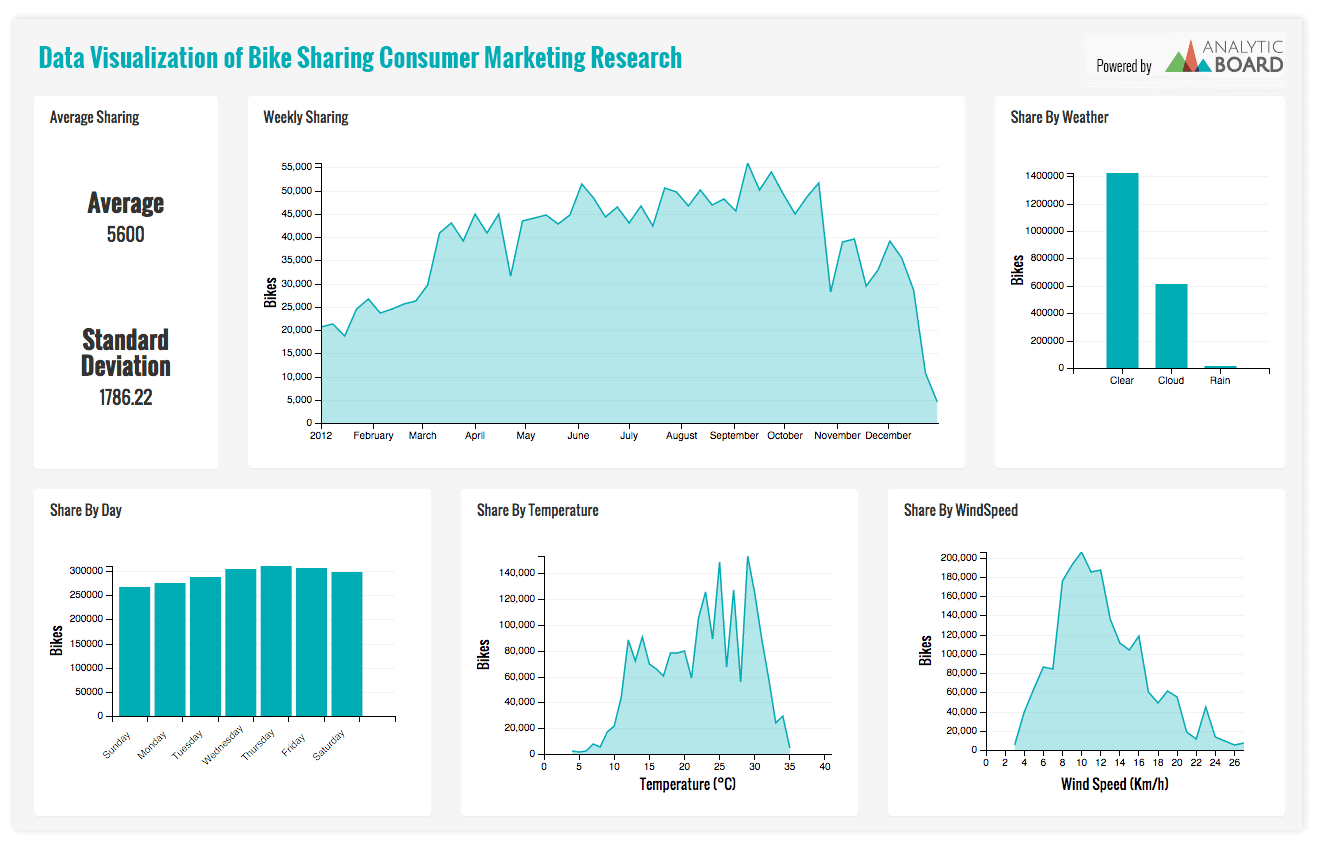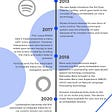# Overview

In this post, I will outline the entire process I used to create a text classifier that classifies tweets into one of three sentiment classes: neutral, positive, and negative.

In terms of overall process, I followed the Cross-Industry Standard for Data Mining, abbreviated CRISP-DM. The CRISP-DM data science process has 6 main stages outlined below:

2. Data Understanding
3. Data Preparation
4. Modeling
5. Evaluation
6. Deployment

Below will go through each of these steps in detail, with the exception of deployment for the purposes of this post.

The overall goal of this process is to create a classifier that can label tweets based on their sentiment (positive, negative, or neutral). This classifier will be created in the context of tracking public sentiment surrounding various product releases / events. Stakeholders for this project are likely product managers / investor and public relations professionals who are invested in how the public is feeling towards newly released products, and various other business proceedings. In conjunction with other tools, companies could use this classifier to create a sentiment score based on a certain number of recent tweets, and track that over time to monitor changes in sentiment over time. Additionally, companies could pull down a batch of tweets at certain times, filtered for various products or topics. If a broad enough sample is used, it could likely help provide good insight into whether consumers are feeling neutral, positive, or negative towards recent releases.

According to https://www.internetlivestats.com/twitter-statistics/, there are over 500 million tweets sent per day. Harnessing public sentiment from this amount of data would undoubtedly be helpful for tech companies looking to track how the public is feeling towards them.

# 2. Data Understanding

The main dataset used throughout this data science process will be coming from CrowdFlower via the following url: `https://data.world/crowdflower/brands-and-product-emotions`.

The following summary of the dataset is provided on CrowdFlower:

Contributors evaluated tweets about multiple brands and products. The crowd was asked if the tweet expressed positive, negative, or no emotion towards a brand and/or product. If some emotion was expressed they were also asked to say which brand or product was the target of that emotion.

As the dataset contains labels classifying the tweet as positive, negative, or neutral, along with detailed tweet text, the dataset available is a good match for our business goals.

To start, begin by importing necessary libraries and begin exploring data.

`# import necessary librariesimport pandas as pdimport numpy as npimport matplotlib.pyplot as plt%matplotlib inlineimport seaborn as snsimport nltkfrom nltk.corpus import stopwordsfrom nltk.collocations import *import stringimport refrom sklearn.metrics import accuracy_score, precision_score, recall_score, f1_scorefrom sklearn.ensemble import RandomForestClassifierfrom sklearn.model_selection import train_test_split, GridSearchCV, RandomizedSearchCV from sklearn.model_selection import cross_val_score, StratifiedKFoldfrom sklearn.feature_extraction.text import TfidfVectorizer, CountVectorizerfrom sklearn.feature_selection import SelectKBest, f_classiffrom sklearn.svm import LinearSVCfrom imblearn.over_sampling import SMOTEfrom keras.preprocessing.sequence import pad_sequencesfrom keras.layers import Input, Dense, LSTM, Embedding, Dropout, Activation, Bidirectional, GlobalMaxPool1Dfrom keras.models import Sequentialfrom keras import initializers, regularizers, constraints, optimizers, layersimport tensorflow# load datasetraw_df = pd.read_csv('judge-1377884607_tweet_product_company.csv', encoding='latin_1')`

Look at other aspects of dataset:

`raw_df.info()# display value countsdisplay(raw_df['emotion_in_tweet_is_directed_at'].value_counts())display(raw_df['is_there_an_emotion_directed_at_a_brand_or_product'].value_counts())`

Unsurprising given the origin of our dataset, the products identified are either Apple or Google products. Looking at sentiment, the majority of entries seem to fall under a neutral sentiment (‘No emotion toward brand or product’), with the next largest group being tagged as ‘Positive emotion’. There is some clear class imbalance present with only 570 entries belonging to the ‘Negative emotion’ class. The lack of negtive sentiment labels is likely a weakness of this dataset.

`# rename columns so easier to work withdf = raw_df.copy()df.columns = ['text', 'product_brand', 'sentiment']df.head()`

Explore potential missing values:

`df.isna().sum()`

Further explore missing values in product_brand column as these are missing the most.

`# display missing values in the product_brand columndf.loc[df['product_brand'].isna()]# display sentiment breakdowns of missing product_brand entriesdf.loc[df['product_brand'].isna()]['sentiment'].value_counts()`

We see that the majority of missing product_brand values are also labeled as no emotion toward brand or product, which makes sense as a lot of the neutral-labeled tweets may not be directed at a specific brand or product, and therefore would be missing a product_brand tagging. Additionally, this column will not be used in our process of tweet classification.

Drop unnecessary columns and handle missing value for additional EDA.

`# drop product_brand columnclean_df = df.drop(['product_brand'], axis=1)# handle missing valuesclean_df = clean_df.dropna(subset=['text'])clean_df.info()`

Next, split dataset into text and class_labels to further explore corpus statistics.

`tweets = clean_df['text']class_labels = clean_df['sentiment']`

Tokenize tweets and print total vocab of our dataset:

`tokenized = list(map(nltk.word_tokenize, tweets.dropna())) raw_tweet_vocab = set()for tweet in tokenized:    raw_tweet_vocab.update(tweet)print(len(raw_tweet_vocab))`

We see there is a vocab size just over 13,000. This is not huge for text classification standards, but it is what we have to work with so we will continue. Print the average tweet length:

`# print average tweet sizemean_tweet_size = []for tweet in tokenized:    mean_tweet_size.append(len(tweet))np.mean(mean_tweet_size)`

We see the average tweet is just over 24 words. Next, let’s look at the most common words / symbols in our dataset:

`# display frequency distribution of raw datasettweets_concat = []for tweet in tokenized:    tweets_concat += tweet    # display the 15 most common wordsunprocessed_freq_dist = nltk.FreqDist(tweets_concat)unprocessed_freq_dist.most_common(25)`

We can also visualize this as a barplot

`# visualize frequency distributiontop_20 = pd.DataFrame(unprocessed_freq_dist.most_common(20), columns=['token', 'freq'])plt.figure(figsize=(10, 5))sns.barplot(x=top_20['token'], y=top_20['freq'], color='purple')plt.xlabel('Token')plt.ylabel('Frequency')plt.title('Most Common Words in Dataset')plt.show()`

From first glance, we can see a number of the top appear words / tokens are stopwords or punctuation. Not surprising given our dataset, we also see the most common tokens are related to twitter tweet structure, with `#` and `@` along with others standing out. For additional EDA processed, we will try removing stopwords to see if additional information can be extracted from the data.

Next, we will build a few functions that will help preprocess and further examine our dataset.

`def initial_tweet_process(tweet, stopwords_list):    """    Function to intially process a tweet to assist in EDA / data understanding.     Input: tweet of type string, stopwords_list of words to remove    Returns: tokenized tweet, converted to lowercase, with all stopwords removed    """    # tokenize    tokens = nltk.word_tokenize(tweet)        # remove stopwords and lowercase    stopwords_removed = [token.lower() for token in tokens if token.lower() not in stopwords_list]        # return processed tweet    return stopwords_removeddef concat_tweets(tweets):    """    Function to concatenate a list of tweets into one piece of text.    Input: tweets (list of tweets)    Returns: concatenated tweet string    """    tweets_concat = []    for tweet in tweets:        tweets_concat += tweet    return tweets_concatdef process_concat(raw_text, stopwords_list):    """    Function to process and return concatenated tweets.  Takes raw text, and stopwords.     Returns: concatenated tweets    """    processed_text = raw_text.apply(lambda x: initial_tweet_process(x, stopwords_list))    return concat_tweets(list(processed_text))def print_normalized_word_freq(freq_dist, n=15):    """    Print a normalized frequency distribution from a given distribution. Returns top n results.     """    total_word_count = sum(freq_dist.values())    top = freq_dist.most_common(n)        print('Word\t\t\tNormalized Frequency')    for word in top:        normalized_freq = word / total_word_count        print('{} \t\t\t {:.4}'.format(word, normalized_freq))        return Nonedef print_bigrams(tweets_concat, n=15):    """    Function takes concatenated tweets and prints most common bigrams    """    bigram_measures = nltk.collocations.BigramAssocMeasures()    finder = BigramCollocationFinder.from_words(tweets_concat)    tweet_scored = finder.score_ngrams(bigram_measures.raw_freq)    display(tweet_scored[:n])    return tweet_scoreddef display_pmi(tweets_concat, freq_filter=10, n=15):    """    Function that takes concatenated tweets and a freq_filter number. Displays PMI scores.     """    bigram_measures = nltk.collocations.BigramAssocMeasures()    tweet_pmi_finder = BigramCollocationFinder.from_words(tweets_concat)    tweet_pmi_finder.apply_freq_filter(freq_filter)    tweet_pmi_scored = tweet_pmi_finder.score_ngrams(bigram_measures.pmi)    display(tweet_pmi_scored[:n])    return tweet_pmi_scored`

Now that we have set up a few functions to display bigrams, PMI scores, etc. we will move on to setting up stopwords and removing these from our dataset. We take advantage of the stopwords provided by nltk

`stopwords_list = stopwords.words('english') + list(string.punctuation)stopwords_list += ["''", '""', '...', '``']stopwords_list += ['mention', 'sxsw', 'link', 'rt', 'quot', 'google', 'apple']`

Next process using our function above:

`concat_all = process_concat(all_tweets['text'], stopwords_list)`

Produce frequency distribution:

`freqdist_all = nltk.FreqDist(concat_all)`

I also chose to repeat the process after separating my dataset by class label. This was done to try and find distinctions between the different class labels. Creating frequency distributions for each split allowed me to create a subplot visualization to make comparison easier.

`# visualize with subplotsfig, axes = plt.subplots(2, 2, figsize=(20, 5))freq_dists = [neutral_top_15, positive_top_15, negative_top_15, ambig_top_15]labels = ['Top 15 (Neutral)', 'Top 15 (Positive)', 'Top 15 (Negative)', 'Top 15 (Ambiguous)']for idx, ax in enumerate(axes.flat):    sns.barplot(data=pd.DataFrame(freq_dists[idx], columns=['token', 'count']),                x='token',                y='count',                ax=ax,                color='purple')    ax.set_title(labels[idx])plt.tight_layout()`

Which produced an output of the following:

Comparing the top words, we see again that the following words appear frequently in all class labels, and are therefore not as helpful in classification. Update stopwords list and reprint frequency distributions. After updated stopwords to further address this, I also produced a breakdown of the top bigrams, and PMI scores for the separated datasets.

`# pull out top n bigramstop_n = 15top_neutral_bigrams = pd.DataFrame(neutral_bigrams[:top_n], columns=['bigram', 'score'])top_positive_bigrams = pd.DataFrame(positive_bigrams[:top_n], columns=['bigram', 'score'])top_negative_bigrams = pd.DataFrame(negative_bigrams[:top_n], columns=['bigram', 'score'])top_ambig_bigrams = pd.DataFrame(ambig_bigrams[:top_n], columns=['bigram', 'score'])# visualizefig, axes = plt.subplots(2, 2, figsize=(20, 10))bigrams = [top_neutral_bigrams, top_positive_bigrams, top_negative_bigrams, top_ambig_bigrams]labels = ['Top 15 (Neutral)', 'Top 15 (Positive)', 'Top 15 (Negative)', 'Top 15 (Ambiguous)']for idx, ax in enumerate(axes.flat):    sns.barplot(data=bigrams[idx],                y='bigram',                x='score',                ax=ax,                color='purple')    ax.set_title(labels[idx])plt.tight_layout()`
`# pull out top n pmi scorestop_n = 15top_neutral_pmi = pd.DataFrame(neutral_pmi[:top_n], columns=['word combo', 'score'])top_positive_pmi = pd.DataFrame(positive_pmi[:top_n], columns=['word combo', 'score'])top_negative_pmi = pd.DataFrame(negative_pmi[:top_n], columns=['word combo', 'score'])# visualizefig, axes = plt.subplots(3, 1, figsize=(20, 10))pmis = [top_neutral_pmi, top_positive_pmi, top_negative_pmi]labels = ['Top 15 (Neutral)', 'Top 15 (Positive)', 'Top 15 (Negative)', 'Top 15 (Ambiguous)']for idx, ax in enumerate(axes.flat):    sns.barplot(data=pmis[idx],                y='word combo',                x='score',                ax=ax,                color='purple')    ax.set_title(labels[idx])plt.tight_layout()`

Looking at PMI scores, we can see some further trends standing out. Some word combinations within the positive dataset that stand out include: (choice, awards), (uberguide, sponsored), (looking, forward). Some word combinations that stood out within the negative set included: (fascist, company) and (design, headaches).

Now that we have a good sense of our data and the distribution, we can move on to the data preparation phase.

# 3. Data Preparation

We will now leverage information learned during the data understanding phase to preprocess the dataset and prepare data for modeling.

`# pull in copy of datasetclean_df = raw_df.copy()# relabel columnsclean_df.columns = ['text', 'product_brand', 'sentiment']# drop product_brand column, handle missing values and duplicatesclean_df = clean_df.drop('product_brand', axis=1)clean_df = clean_df.dropna()clean_df = clean_df.drop_duplicates()# remove ambiguous tweetsclean_df = clean_df.loc[clean_df['sentiment'] != "I can't tell"]# separate dataset into text and class_labelstext = clean_df['text']class_labels = clean_df['sentiment']`

Now we have re-pulled in the dataset, we can separate into training and test sets so we have a hold-out set to validate our results against.

`# split tweets and labels into train and test sets for validation purposesX_train, X_test, y_train, y_test = train_test_split(text, class_labels, stratify=class_labels, random_state=SEED)`

Create function to preprocess tweets:

`def preprocess_tweet(tweet, stopwords_list):    """    Function to preprocess a tweet.     Takes: tweet, stopwords list    Returns: processed tweet with stopwords removed and converted to lowercase    """    processed = re.sub("\'", '', tweet) # handle apostrophes    processed = re.sub('\s+', ' ', processed) # handle excess white space    tokens = nltk.word_tokenize(processed)    stopwords_removed = [token.lower() for token in tokens if token.lower() not in stopwords_list]    return ' '.join(stopwords_removed)`

Next preprocess our tweets and vectorize our dataset using both a count vectorizer and TF-IDF vectorizer. This will enable us to compare results between the two vectorization methods to see if one is better than the other.

`# preprocess train and test setsX_train_preprocessed = X_train.apply(lambda x: preprocess_tweet(x, updated_stopwords))X_test_preprocessed = X_test.apply(lambda x: preprocess_tweet(x, updated_stopwords))# create vectorizers with unigram and bigramscount_vectorizer = CountVectorizer(ngram_range=(1,2), analyzer='word') # use unigrams and ngramstfidf_vectorizer = TfidfVectorizer(ngram_range=(1,2), analyzer='word') # use unigrams and ngrams# fit to preprocessed dataX_train_count = count_vectorizer.fit_transform(X_train_preprocessed)X_test_count = count_vectorizer.transform(X_test_preprocessed) X_train_tfidf = tfidf_vectorizer.fit_transform(X_train_preprocessed) X_test_tfidf = tfidf_vectorizer.transform(X_test_preprocessed)`

In addition to count vectorizing and TF-IDF vectorizing, we will also take advantage of Global Vectors for Word Representation (GloVe). More information can be found here: https://nlp.stanford.edu/projects/glove/

`# tokenize datasetstokenized_X_train = X_train_preprocessed.map(nltk.word_tokenize).valuestokenized_X_test = X_test_preprocessed.map(nltk.word_tokenize).values# get total training vocabulary sizetotal_train_vocab = set(word for tweet in tokenized_X_train for word in tweet)train_vocab_size = len(total_train_vocab)print(f'There are {train_vocab_size} unique tokens in the processed training set.')def glove_vectors(vocab):    """    Returns appropriate vectors from GloVe file.    Input: vocabulary set to use.    """    glove = {}    with open('glove.6B.50d.txt', 'rb') as f:        for line in f:            parts = line.split()            word = parts.decode('utf-8')            if word in vocab:                vector = np.array(parts[1:], dtype=np.float32)                glove[word] = vector    return gloveglove = glove_vectors(total_train_vocab)class W2vVectorizer(object):        def __init__(self, w2v):        # Takes in a dictionary of words and vectors as input        self.w2v = w2v        if len(w2v) == 0:            self.dimensions = 0        else:            self.dimensions = len(w2v[next(iter(glove))])        # Note: Even though it doesn't do anything, it's required that this object implement a fit method or else    # it can't be used in a scikit-learn pipeline      def fit(self, X, y):        return self                def transform(self, X):        return np.array([            np.mean([self.w2v[w] for w in words if w in self.w2v]                   or [np.zeros(self.dimensions)], axis=0) for words in X])`

As can be seen above, I followed the method outlined by Flatiron School and built a class for the w2v vectorization process. Once this is done, data can be vectorized with this method:

`# instantiate vectorizer objects with glovew2v_vectorizer = W2vVectorizer(glove)# transform training and testing dataX_train_w2v = w2v_vectorizer.transform(tokenized_X_train)X_test_w2v = w2v_vectorizer.transform(tokenized_X_test)`

Now that our datasets are vectorized, we can move on to the modeling phase.

# 4. Modeling

This is a classification task, tasked with classifying the sentiment of tweets based on the text within the tweet. Three primary models will be relied on for classification:
1. Random Forests
2. Linear SVM
3. Neural Networks

Overfitting will be addressed thru hyperparameter tuning and dropout layers in the case of neural networks.

This is a multi-class classification problem, with three available class labels (Neutral, Positive, or Negative). As a result, the performance metric we will focus on throughout this process will be accuracy. We are not too concerned about the ramifications of false positives or false negatives. For this reason accuracy will be our selected performance metric.

`# instantiate random forest classifiers, with balanced class_weightrf_count = RandomForestClassifier(random_state=SEED, n_jobs=-1, class_weight='balanced')rf_tfidf = RandomForestClassifier(random_state=SEED, n_jobs=-1, class_weight='balanced')rf_w2v = RandomForestClassifier(random_state=SEED, n_jobs=-1, class_weight='balanced')# fit to training setsrf_count.fit(X_train_count, y_train)rf_tfidf.fit(X_train_tfidf, y_train)rf_w2v.fit(X_train_w2v, y_train)`

Now get scores of vectorized data:

`# Count Vectorizedcount_train_score = rf_count.score(X_train_count, y_train)count_test_score = rf_count.score(X_test_count, y_test)print(f'Count Vectorized Train Score: {count_train_score}')print(f'Count Vectorized Test Score: {count_test_score}')print('--------')# TF-IDF Vectorizedtfidf_train_score = rf_tfidf.score(X_train_tfidf, y_train)tfidf_test_score = rf_tfidf.score(X_test_tfidf, y_test)print(f'TF-IDF Vectorized Train Score: {tfidf_train_score}')print(f'TF-IDF Vectorized Test Score: {tfidf_test_score}')print('--------')# W2V Vectorizedw2v_train_score = rf_w2v.score(X_train_w2v, y_train)w2v_test_score = rf_w2v.score(X_test_w2v, y_test)print(f'Word2Vec Vectorized Train Score: {w2v_train_score}')print(f'Word2Vec Vectorized Test Score: {w2v_test_score}')`

Reviewing baseline random model scores for our three vectorized datasets (count, tf-idf, and word2vec using glove), we can see that results are fairly consistent across our vectorization methods. Further, looking at our high training set accuracy score vs. test scores, shows we are likely overfitting slightly to the training data. I decided to address overfitting through hyper parameter tuning using grid search.

`# set paramsgrid_search_params = {    'min_samples_split': [4, 5],    'min_samples_leaf': [3, 4],    'max_depth': [25, 50, 75],    'max_features': ['auto', 'sqrt'],    'bootstrap': [True, False],    'criterion': ['entropy', 'gini']}# instantiate classifierrf_classifier = RandomForestClassifier(n_jobs=-1, random_state=SEED, class_weight='balanced', n_estimators=100)# instantiate grid searchrf_gs_count = GridSearchCV(estimator=rf_classifier,                            param_grid=grid_search_params,                            cv=3,                            scoring='accuracy',                            return_train_score=True,                           verbose=1)# fit to count vectorizedrf_gs_count.fit(X_train_count, y_train)# print count-vectorized resultsmean_train_score_count = np.mean(rf_gs_count.cv_results_['mean_train_score'])mean_test_score_count = np.mean(rf_gs_count.cv_results_['mean_test_score'])print(f'Random Search Train Accuracy (Count Vect.): {mean_train_score_count}')print(f'Random Search Test Accuracy (Count Vect.): {mean_test_score_count}')# display best paramsrf_gs_count.best_params_`

Looking at tuned model scores, we can see that overfitting has been largely addressed, but testing performance is not looking great. The cross-validated results are showing ~56.5% accuracy score on the testing set. Given a balanced dataset, a simple model would get roughly 33% accuracy through random guessing, so our model is outperforming those metrics.

I will not present the process here, but this was repeated for the two other vectorization strategies. Moving on to SVC we will see the process is largely the same.

`# create linear SVCsvc_count = LinearSVC(random_state=SEED, class_weight='balanced', max_iter=5000)svc_tfidf = LinearSVC(random_state=SEED, class_weight='balanced', max_iter=5000)svc_w2v = LinearSVC(random_state=SEED, class_weight='balanced', max_iter=5000)# fit to training setssvc_count.fit(X_train_count, y_train)svc_tfidf.fit(X_train_tfidf, y_train)svc_w2v.fit(X_train_w2v, y_train)`

Get baseline scores:

`# Count Vectorizedcount_train_score = svc_count.score(X_train_count, y_train)count_test_score = svc_count.score(X_test_count, y_test)print(f'Count Vectorized Train Score: {count_train_score}')print(f'Count Vectorized Test Score: {count_test_score}')print('-----')# TF-IDF Vectorizedtfidf_train_score = svc_tfidf.score(X_train_tfidf, y_train)tfidf_test_score = svc_tfidf.score(X_test_tfidf, y_test)print(f'TF-IDF Vectorized Train Score: {tfidf_train_score}')print(f'TF-IDF Vectorized Test Score: {tfidf_test_score}')print('-----')# Word2Vec Vectorizedw2v_train_score = svc_w2v.score(X_train_w2v, y_train)w2v_test_score = svc_w2v.score(X_test_w2v, y_test)print(f'Word2Vect Vectorized Train Score: {w2v_train_score}')print(f'Word2Vect Vectorized Test Score: {w2v_test_score}')`

Looking at baseline LinearSVC results we can see that, similar to initial random forest models, we are overfitting to training data, except with word2vec vectorized data. Move forward with hyperparameter tuning to try and improve results / address overfitting. Again this was performed using gridsearch.

`# set params for grid searchsvc_params = {    'C': [0.00001, 0.0001, .001],    'loss': ['hinge', 'squared_hinge']}# grid search svc_classifier = LinearSVC(random_state=SEED, class_weight='balanced', max_iter=10000)svc_gs_count = GridSearchCV(svc_classifier,                            svc_params,                            return_train_score=True,                            scoring='accuracy',                            verbose=1)# fit to training datasvc_gs_count.fit(X_train_count, y_train)# print count-vectorized grid-search resultsmean_train_score_count = np.mean(svc_gs_count.cv_results_['mean_train_score'])mean_test_score_count = np.mean(svc_gs_count.cv_results_['mean_test_score'])print(f'Grid Search Train Accuracy (Count Vect.): {mean_train_score_count}')print(f'Grid Search Test Accuracy (Count Vect.): {mean_test_score_count}')# display best paramssvc_gs_count.best_params_`

We can see that overfitting again has largely been addressed. Additionally, results are looking slightly better than our random forest results. After applying the same methodology to the other vectorization strategies, we can compare all the results. Next up, is building out neural networks.

`# convert labels to one-hot encoded formaty_train_encoded = pd.get_dummies(y_train).valuesy_test_encoded = pd.get_dummies(y_test).values# set up last layer of neural network for multi-class classification with 3 labelslast_layer_activation = 'softmax' last_layer_units = 3`

Next, build the model:

`model = Sequential()model.add(Dropout(rate=0.5, input_shape=input_shape))model.add(Dense(units=50, activation='relu'))model.add(Dropout(rate=0.5))model.add(Dense(units=50, activation='relu'))model.add(Dropout(rate=0.5))model.add(Dense(units=last_units, activation=last_activation))`

Compile the model:

`model.compile(loss='categorical_crossentropy',                  optimizer='adam',                  metrics=['accuracy'])`

Display model summary

`display(model.summary())`

Which gives something like this:

Next fit to training data:

`count_model.fit(X_train_count, y_train_encoded,                 epochs=10,                batch_size=100,                validation_split=0.2)`

Running will produce output similar to the following:

Looking at the results, we can see overfitting starting to take place around epoch 4. We will keep this in mind as we reproduce these results to land on our best neural network model. After this is reproduced for all vectorization strategies, we can compare all model results.

# 5. Evaluation

Reprinting all accuracy scores for both training and test sets we can compare our different models:

`# summarize training and test scores for all models run:best_rf_models = [best_rf_count, best_rf_tfidf, best_rf_w2v]best_svc_models = [best_svc_count, best_svc_tfidf, best_svc_w2v]print(f'Best Tuned Random Forest (Count Vectorized) Test Accuracy: {best_rf_count.score(X_test_count, y_test)}')print(f'Best Tuned Random Forest (Count Vectorized) Train Accuracy: {best_rf_count.score(X_train_count, y_train)}')print(f'Best Tuned Random Forest (TF-IDF Vectorized) Test Accuracy: {best_rf_tfidf.score(X_test_tfidf, y_test)}')print(f'Best Tuned Random Forest (TF-IDF Vectorized) Train Accuracy: {best_rf_tfidf.score(X_train_tfidf, y_train)}')print(f'Best Tuned Random Forest (Word2Vec) Test Accuracy: {best_rf_w2v.score(X_test_w2v, y_test)}')print(f'Best Tuned Random Forest (Word2Vec) Train Accuracy: {best_rf_w2v.score(X_train_w2v, y_train)}')print(f'Best Tuned LinearSVC (Count) Test Accuracy: {best_svc_count.score(X_test_count, y_test)}')print(f'Best Tuned LinearSVC (Count) Train Accuracy: {best_svc_count.score(X_train_count, y_train)}')print(f'Best Tuned LinearSVC (TF-IDF) Test Accuracy: {best_svc_tfidf.score(X_test_tfidf, y_test)}')print(f'Best Tuned LinearSVC (TF-IDF) Train Accuracy: {best_svc_tfidf.score(X_train_tfidf, y_train)}')print(f'Best Tuned LinearSVC (w2v) Test Accuracy: {best_svc_w2v.score(X_test_w2v, y_test)}')print(f'Best Tuned LinearSVC (w2v) Train Accuracy: {best_svc_w2v.score(X_train_w2v, y_train)}')print(f'Best Neural Network (Count) Test Accuracy: {nn_count_test_score}')print(f'Best Neural Network (Count) Train Accuracy: {nn_count_train_score}')print(f'Best Neural Network (TF-IDF) Test Accuracy: {nn_tfidf_test_score}')print(f'Best Neural Network (TF-IDF) Train Accuracy: {nn_tfidf_train_score}')print(f'Best Neural Network (Word2Vec) Test Accuracy: {nn_w2v_test_score}')print(f'Best Neural Network (Word2Vec) Train Accuracy: {nn_w2v_train_score}')`

We can also visualize as a barplot to make it easier to see:

`# visualize testing scorestest_labels = pd.Series(['RF (Count)',                         'RF (TF-IDF)',                         'RF (Word2Vec)',                         'LinearSVC (Count)',                         'LinearSVC (TF-IDF)',                         'LinearSVC (Word2Vec)',                         'Neural Net (Count)',                         'Neural Net (TF-IDF)',                         'Neural Net (Word2Vec)'])test_acc = pd.Series([best_rf_count.score(X_test_count, y_test),                      best_rf_tfidf.score(X_test_tfidf, y_test),                      best_rf_w2v.score(X_test_w2v, y_test),                      best_svc_count.score(X_test_count, y_test),                      best_svc_tfidf.score(X_test_tfidf, y_test),                      best_svc_w2v.score(X_test_w2v, y_test),                      nn_count_test_score,                      nn_tfidf_test_score,                      nn_w2v_test_score])plt.figure(figsize=(15, 5))sns.barplot(x=test_labels, y=test_acc, color='grey')plt.tight_layout()plt.show()`

After running, we can see the best model we have seen, according to testing accuracy is our neural network on count vectorized data.

--

--

--

Love podcasts or audiobooks? Learn on the go with our new app.

## Dynamic Futures Portfolio Under a Multifactor Gaussian Framework## Visualize, Understand, and Act:## Responsible AI in Action## Covid mortality analysis## What is data visualization and why is it important?## D4S Sunday Briefing #155## How to correctly select a sample from a huge dataset in machine learning## The Evolution of in-Car Voice Control Leads to Win-Win for All## Automated Library KerasTuner for Hyperparameter Tuning of ML and DL Models## Automated Reply Suggestion Using Natural Language Processing Techniques## An intro about evolutionary algorithms